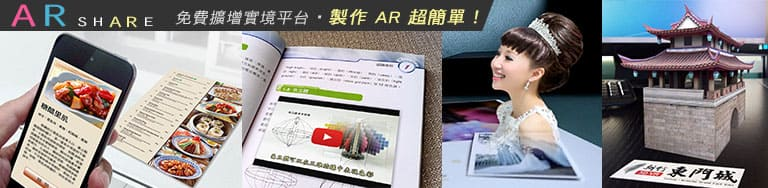# Unity Script 常用語法教學(unity課程入門學習筆記)

● 在 Console 面板輸出訊息
// print("<color=red>這樣能輸出紅色訊息</color>");

● 鍵盤按鍵觸發 (通常寫在 Update 中)
if ( Input.GetKey("a") ){　}　// A 鍵被按住時持續觸發(每個 frame 執行一次)
if ( Input.GetKeyDown("a") ){　}　// A 鍵被按下瞬間觸發一次
if ( Input.GetKeyUp("a") ){　}　// A 鍵被放開瞬間觸發一次
※ 請參閱 鍵盤控制物體移動、旋轉 實作範例

● 滑鼠按鍵觸發 (通常寫在 Update 中)
if ( Input.GetMouseButton(0) ){　}　// 滑鼠左鍵被按住時持續觸發(每個 frame 執行一次)
if ( Input.GetMouseButtonDown(0) ){　}　// 滑鼠左鍵被按下瞬間觸發一次
if ( Input.GetMouseButtonUp(0) ){　}　// 滑鼠左鍵被放開瞬間觸發一次
0、1、2 分別代表滑鼠 左、右、中 鍵● Transform 相關
transform.Translate( x, y, z );　// 移動
transform.Rotate( x, y, z );　// 旋轉
transform.position = new Vector3( x, y, z );　// 設定位置(世界座標)
transform.localPosition = new Vector3( x, y, z );　// 設定位置(相對於母物體)
transform.eulerAngles = new Vector3( x, y, z );　// 設定角度
transform.localEulerAngles = new Vector3( x, y, z );　// 設定角度(相對於母物體)
transform.LookAt( otherGameObject.transform );　// 讓物件的 Z 軸朝(指)向另一個物件的軸心

transform.forward　// 代表物件的 z 軸方向( unity以z軸為前方，建模時最好符合此規則，讓z軸朝前。)
例如要讓物件朝著另一個物件的前面(z 軸方向)移動：
transform.Translate ( otherGameObject.transform.forward );

● 設定與解除連結(階層關係)
A.transform.parent = B.transform;　// 設定 B 為 A 的母物體
A.transform.parent = null;　// 解除 A 的母物體

● 抓取物件、元件
請參閱「unity 抓取物件(GameObject)和元件(Component)」這篇文章。

● 啟用(或停用)物件、元件
yourGameObject.SetActive( true );　// 啟用物件
yourComponent.enabled = true;　// 啟用元件

● 複製、刪除物件
Instantiate( yourObject );　// 動態產生 object
Destroy( yourObject );　// 刪除 object

● 呼叫其他物件的函數
GameObject.Find("物件名稱").GetComponent<script名稱>().函數名稱();
被呼叫函數的前面要加 public，例如： public void abc(){ }

● 抓取其他 script 的變數(例如要在 script_A 抓取 script_B 的變數)
在 script_B 宣告變數時，前面加上 static public (例如 static public int a )，
之後在 script_A 打 script_B.a ，即可取得 script_B 的變數值。

● 抓取(設定) Animator 中的參數(Parameter)
GetComponent<Animator>().GetBool( "參數名稱" );　// 取得參數的布林值
GetComponent<Animator>().SetBool( "參數名稱", 值 );　// 設定參數的布林值
把 Bool 改成 Integer 就是整數、改成 Float 就是浮點數數

● 定時呼叫函數 (常用來製作計時器)
Invoke("函數名稱", 2);　// 2 秒後呼叫函數
InvokeRepeating("函數名稱", 1.5f, 0.3f);　// 1.5 秒後呼叫函數，之後每隔 0.3 秒都會呼叫函數
以上兩者的差別在於 Invoke 只會呼叫一次，而 InvokeRepeating 會重複呼叫。
CancelInvoke();　// 停止(取消)定時呼叫

● 物理學(Physics)
Physics.gravity = new Vector3(0, -9.81f, 0);　// 設定重力
GetComponent<Rigidbody>().AddForce( x, y, z, 力量模式 );
// 對 Rigidbody 施加力量，(x,y,z)為世界座標，代表力量的強度和方向
// 力量模式填 ForceMode.Force 適用於持續的力、填 ForceMode.Impulse 適用於瞬間的力

● 物件碰撞偵測
請參閱「unity Collision Trigger 碰撞偵測與觸發對應表」這篇文章。

● 重新開始、結束遊戲
場景索引號可在 Build Setting 視窗查看、script 開頭要加 using UnityEngine.SceneManagement;
Application.Quit();　// 退出遊戲、關閉 app

● 一些 MonoBehaviour 常用的函數
void Start(){}　// 只有第一個 frame 會呼叫
void Update(){}　// 每個 frame 都會呼叫
void OnMouseDown(){}　// 套用 script 的 collider 物件被滑鼠點擊瞬間會呼叫

● 材質、貼圖相關
public Texture2D myPic;
GetComponent<Renderer>().material.mainTexture = myPic;　// 設定材質的主要貼圖
GetComponent<Renderer>().material.color = new Color( r, g, b );
// 設定材質色彩( RGB 值為 0~1 )

◎ Random.Range( min, max );　// 產生亂數(若都填整數，回傳值最大只會是 max-1)
◎ GetComponent<RectTransform>().sizeDelta = new Vector2 ( x, y );
// 設定 RectTransform (UI物件)的寬、高※ 您必需先登入，才能填寫送出。